Introduction To Electric Circuits - 9 Edition - Chapter 3 - Problem Dp 3-6
Register Now

Join StudySoup

Get Full Access to Introduction To Electric Circuits - 9 Edition - Chapter 3 - Problem Dp 3-69781118477502

# The input to the circuit shown in Figure DP 3-6 is the current source current is. The

Introduction to Electric Circuits | 9th Edition

Problem DP 3-6

The input to the circuit shown in Figure DP 3-6 is the current source current is. The output is the current io. The output is related to the input by io R1 R1 R2 is gis The output of the current divider is proportional to the input. The constant of proportionality g is called the gain of the current divider and is given by g R1 R1 R2 The power supplied by the current source is p vsis is R1R2 R1 R2 is R1R2 R1 R2 is 2 Rinis 2 where Rin R1R2 R1 R2 is called the input resistance of the current divider. (a) Design a current divider to have a gain, g 0.65. (b) Design a current divider to have a gain, g 0.65, and an input resistance, Rin 10000 V. R2 is vs R1 io + Figure DP 3-6

Accepted Solution
Step-by-Step Solution:

Step 1 of 4

a)

Given that ,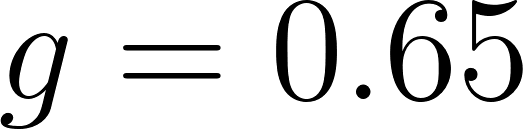We need to design a current divider .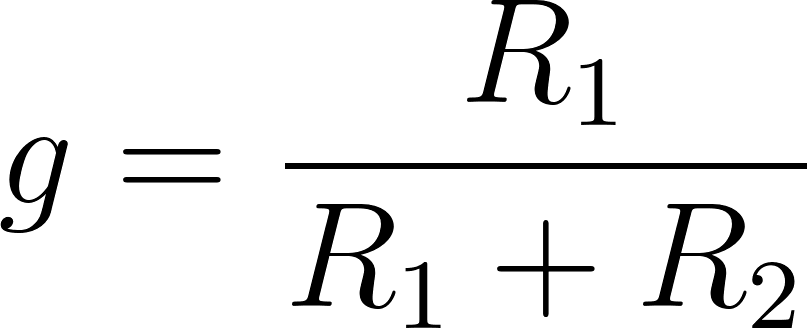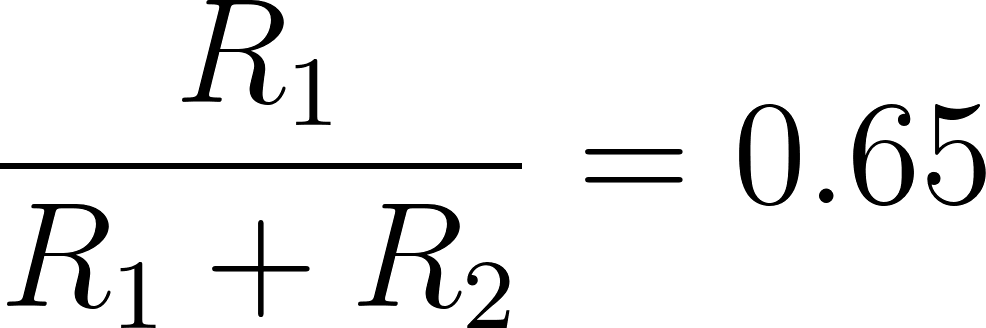Assuming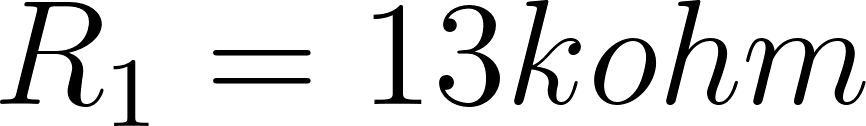since one equation with two unknowns.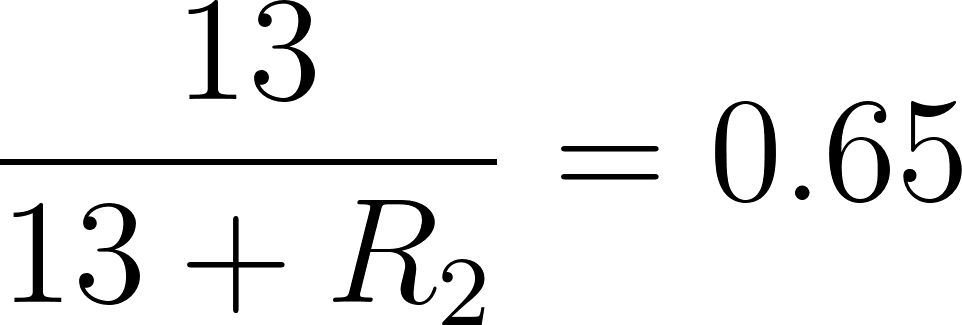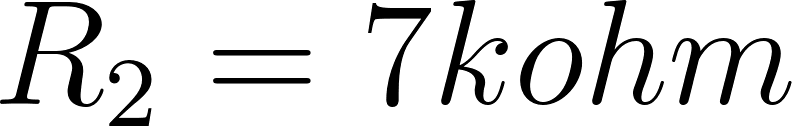###### Chapter 3, Problem DP 3-6 is Solved

Step 2 of 4

Step 3 of 4

Unlock Textbook Solution